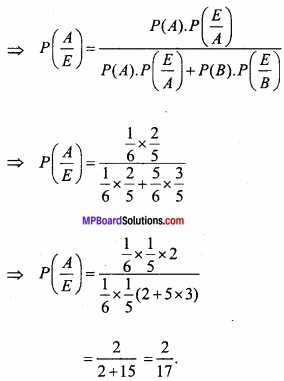# MP Board Class 12th Maths Important Questions Chapter 13 Probability

## MP Board Class 12th Maths Important Questions Chapter 13 Probability

### Probability Important Questions

Probability Objective Type Questions

Question 1.

Question 1.
A bag contains 5 brown and 4 white socks. A man drawn two socks from the bag. The probability that both are of the same colour is:
(a) $$\frac { 5 }{ 108 }$$
(b) $$\frac { 18 }{ 108 }$$
(c) $$\frac { 30 }{ 108 }$$
(d) $$\frac { 48 }{ 108 }$$
(d) $$\frac { 48 }{ 108 }$$

Question 2.
A die is roiled three times. The probability of getting a larger number then the previous number each time is:
(a) $$\frac { 5 }{ 72 }$$
(b) $$\frac { 5 }{ 54 }$$
(c) $$\frac { 13 }{ 216 }$$
(d) $$\frac { 1 }{ 18 }$$
(d) $$\frac { 1 }{ 18 }$$

Question 3.
It is given that the events A and B are such that P(A) = $$\frac{1}{4}$$, P( $$\frac{A}{B}$$ ) = $$\frac{1}{2}$$ and P ( $$\frac{B}{A}$$ ) = $$\frac{2}{3}$$, then P(B) is:
(a) $$\frac{1}{3}$$
(b) $$\frac{2}{3}$$
(c) $$\frac{1}{2}$$
(d) $$\frac{1}{6}$$
(a) $$\frac{1}{3}$$Question 4.
A coin is tossed 4 times. Probability of getting at least one head is:
(a) $$\frac{1}{16}$$
(b) $$\frac{2}{16}$$
(c) $$\frac{14}{16}$$
(d) $$\frac{15}{16}$$
(d) $$\frac{15}{16}$$

Question 5.
The probability of A speaking a truth is $$\frac{4}{5}$$ and that of B speaking a truth is $$\frac{3}{4}$$ The probability that they will contradict each other in answering a fact is:
(a) $$\frac{3}{10}$$
(b) $$\frac{1}{4}$$
(c) $$\frac{7}{20}$$
(d) $$\frac{2}{5}$$
(c) $$\frac{7}{20}$$

Question 2.
Fill in the blanks:

1. P( $$\frac { A∪B }{ C }$$ ) = P ( $$\frac{A}{C}$$ ) + ……………………….
2. P(A∩B) = ………………….. or ………………………….
3. If A and B are two independent events then, P (A ∩ B) = ………………………………..
4. If A and B are two independent events then, P ( $$\frac{A}{B}$$ ) = ……………………………….
5. If the probability of random variable X is P (X), then mean E (X) will be ……………………………..
6. If probability of random variable X is P(X), then variance Var (X) will be ……………………………

1. P( $$\frac{B}{C}$$ ) – P ( $$\frac { A∩B }{ C }$$ )
2. P(B).P ( $$\frac{A}{B}$$ ) or P(A) . P ( $$\frac{B}{A}$$ )
3. P(A).P(B)
4. P(A), P(B) ≠ 0
5. ΣX.P(X)
6. ΣX2.P(X) – [ΣX.P(X)]2.

Question 3.
Write True/False:

1. If A and B are any two events, then P (A – B) = P(A) – P (A∩B).
2. If A and B are any two events, then P (A ∪ B) – P (A∩B) = P(A) + P(B).
3. P( $$\frac{A}{B}$$ ) = $$\frac { P(A∩B) }{ P(A) }$$.
4. If A and B are any two events of a sample space S and F is another event such that P(F) ≠ 0, then P ( $$\frac { P(A∪B) }{ P(F) }$$ ) = P ( $$\frac{A}{F}$$ ) + P ( $$\frac{B}{F}$$ ) – P ( $$\frac { P(A∪B) }{ P(F) }$$ )
5. For any two events A and B. P (A∪B) = P (A∩B ) + P ( $$\bar { A }$$ ∩ B) + P (A ∩$$\bar { B }$$ )

1. True
2. False
3. False
4. True
5. True.Question 4.
Write the answer in one word/sentence :

1. Find the probability that a leap year has 53 Friday.
2. A coin is tossed 4 times. Find the probability of getting at least one head.
3. It is given that the events A and B are such that P (A) = $$\frac{1}{4}$$, P ( $$\frac{A}{B}$$ ) = $$\frac{1}{2}$$ and P ( $$\frac{B}{A}$$ ) = $$\frac{2}{3}$$, then find the value of P(B).
4. There are 5 letters and 5 addressed envelops. If the letters are placed at random what is the probability that exactly 3 letters are placed in right envelops?
5. A problem in mathematics is given to 3 students whose chance of solving it are $$\frac{1}{2}$$, $$\frac{1}{3}$$, $$\frac{1}{4}$$. What is the probability that the problem is solved?

1. $$\frac{2}{7}$$
2. $$\frac{15}{16}$$
3. $$\frac{1}{3}$$
4. $$\frac{1}{12}$$
5. $$\frac{3}{4}$$

Question 1.
(A) Two dice are thrown simultaneously. Find the probability of getting a sum?
Solution:
When two dice are thrown simultaneously.
n(S) = 62 = 36.
Sample space of getting a sum 8 is
A = {(2, 6); (6, 2); (5, 3); (3, 5); (4, 4)}
∴ n(A) = 5
∴ Required probability
P(A) = $$\frac { n(A) }{ n(S) }$$ = $$\frac{5}{36}$$.

(B) Two cubical dice are thrown simultaneously. Find the probability of getting a sum of 9?
Solution:
Solve as Q.No. 1 (A)

(C) Two cubical dice are thrown simultaneously. Find the probability of getting a sum of 7?
Solve as Q.No. 1 (A)

Question 2.
The odds against happening of an event is 3 : 4? Find the probability of its failing?
Solution:
The odds against the happening of an event = 3 : 4
∵ Probability of its failing P ( $$\bar { A }$$ ) = $$\frac { b }{ a+b }$$
⇒ P ( $$\bar { A }$$ ) = $$\frac { 4 }{ 3+4 }$$ = $$\frac { 4 }{ 7 }$$.Question 3.
A card is drawn from a pack of 52 cards. Find the probability that its face?
Solution:
Here, n(S) = 52
∵Cards having face = 4 knave + 4 queens + 4 kings
∴ n(A) = 4 + 4 + 4 = 12
Required probability P(A) = $$\frac { 12 }{ 52 }$$ = $$\frac { 3 }{ 13 }$$

Question 4.
What is the probability that a leap year selected at random will contain 53 Sundays?
Solution:
A leap year consists of 366 days. It has 52 complete weeks and 2 days extra. The equally likely cases for the occurrence of these extra days are:

1. Monday and Tuesday,
2. Tuesday and Wednesday,
3. Wednesday and Thursday,
4. Thursday and Friday,
5. Friday and Saturday,
6. Saturday and Sunday,
7. Sunday and Monday.

Out of these 7 exclusive cases, the last two cases are favourable.
∴ n(S) = 7 and n(E) = 2
∴ The required probability = $$\frac { n(E) }{ n(S) }$$ = $$\frac{2}{7}$$.

Question 5.
The probability of the horse A of winning a race is $$\frac{1}{4}$$ and the probability of the horse B winning the same race is $$\frac{1}{8}$$? What is the probability that one of the horse will win the race?
Solution:
Given, P(A) = Probability of winning the horse A = $$\frac{1}{4}$$
and P(B) = Probability of winning the horse B = $$\frac{1}{8}$$
Since, the events of winning the race by A and B are mutually exclusive, therefore
P(A∪B)= P(A) + P(B)
$$\frac{1}{4}$$ + $$\frac{1}{8}$$ = $$\frac{2+1}{8}$$ = $$\frac{3}{8}$$.Question 6.
(A) A card is drawn from an ordinary pack of cards, find the probability of getting a king or a spade?
Solution:
Let the event A denotes the event of drawing a king and B of drawing a spade. Then we
n(S) = 52, n(A) = 4, n(B) = 13, n(A∩B) = 1
[Since there are 4 kings in pack of cards the cards of spade are 13 includes its king]
∴ P(A) = $$\frac { n(A) }{ n(S) }$$ = $$\frac{4}{52}$$, P(B) = $$\frac { n(B) }{ n(S) }$$ = $$\frac{13}{52}$$
P(A∩B) = $$\frac{1}{52}$$
∴ P(A∪B)= P(A) + P(B) = P(A∩B )
= $$\frac{4}{52}$$ + $$\frac{13}{52}$$ – $$\frac{1}{52}$$ = $$\frac{16}{52}$$ = $$\frac{4}{13}$$.

(B) A card is drawn from a pack of cards, find the probability that neither it is an ace nor a King?
Solution:
Total No. of King = 4, No. of ace = 4
And drawing one card from 8 cards = 8C1
And drawing one card from 52 cards = 52C1
∴ P(A) = $$\frac { ^{ 8 }{ C_{ 1 } } }{ ^{ 52 }{ C_{ 1 } } }$$
∴ Probability of neither ace nor king = P( $$\bar { A }$$ ) = 1 – P(A)
= 1 – $$\frac{2}{13}$$ = $$\frac{11}{13}$$

Question 7.
(A) A dice is thrown once. Find the probability of getting even No. “or ” No. less than 5?
Solution:
When a dice is thrown once the sample space is
S = {1, 2, 3, 4, 5, 6}
n(S) = 6
E1 = {2,4,6}, [∴ n(E1) = 3]
E1 = {1, 2, 3, 4}, [∴ n(E2) = 4]
E1∩E2= {2, 4}, [∴ n(E1 ∩E2)
= $$\frac { n(E_{ 1 }) }{ n(S) }$$ + $$\frac { n(E_{ 2 }) }{ n(S) }$$ – $$\frac { n(E_{ 1 }\cap E_{ 2 }) }{ n(S) }$$
= $$\frac{3}{6}$$ + $$\frac{4}{6}$$ – $$\frac{2}{6}$$ = $$\frac{7-2}{6}$$ = $$\frac{5}{6}$$.(B) A pair of dice is thrown. Find the probability that the sum is not 9 or 11?
Solution:
Total number of ways in which 2 dice can be thrown = 6 × 6 = 36
n(S) = 36
Event of getting sum 9 = {(3, 6), (4, 5), (5, 4), (6, 3)}
Total No. = 4.
∴ Probability of getting sum 9 = $$\frac{4}{36}$$ = $$\frac{1}{9}$$
Now event of getting sum 11 = {(5, 6), (6, 5)}
∴ Probability of getting sum 11 = $$\frac{2}{36}$$ = $$\frac{1}{18}$$
Now probability of getting sum 9 or 11
P(A) = $$\frac{1}{9}$$ + $$\frac{1}{18}$$ = $$\frac{2+1}{18}$$ = $$\frac{3}{18}$$ = $$\frac{1}{6}$$
∴ probability of not getting sum 9 or 11
P( $$\bar { A }$$ ) = 1 – P(A)
= 1 – $$\frac{1}{6}$$ = $$\frac{6 – 1}{6}$$ = $$\frac{5}{6}$$.

Question 8.
If 3 coins are tossed simultaneously, then find the probability of getting at least one head?
Solution:
Here, n(S) = 23 = 8
If $$\bar { A }$$ A denotes not getting a head then A = {{T, T, T)}
∴ n( $$\bar { A }$$ ) = 1
Probability of not getting a head P( $$\bar { A }$$ ) = $$\frac { n(\bar { A) } }{ n(S) }$$ = $$\frac{1}{8}$$
∴ Probability of getting at least one head P(A) = 1 – P( $$\bar { A }$$ )
= 1 – $$\frac{1}{8}$$ = $$\frac{7}{8}$$

Question 9.
A coin is tossed twice. Find the probability of getting head, both the times?
Solution:
Let the event of getting head in first throw be E2 and the second throw be E2.
∴ P(E1) = $$\frac{1}{2}$$ = P(E2) = $$\frac{1}{2}$$
Both the events are independent
∴ P(E1 ∩ E2) = P(E1) × P(E2)
= $$\frac{1}{2}$$ × $$\frac{1}{2}$$
∴ Probability of getting head both the times = P(E1 ∩ E2)
= $$\frac{1}{4}$$Question 10.
In a given race, the odds in favour of three horses A, B and C are 1 : 2, 1 : 3 and 1 : 4. Find the chance that one of them will win the race?
Solution:
The probability of winning the horse, A = $$\frac { 1 }{ 1+2 }$$ = $$\frac { 1 }{ 3 }$$
P(B) = The probability of winning the horse, B = $$\frac { 1 }{ 1+3 }$$ = $$\frac { 1 }{ 4 }$$
P(C) = The probability of winning the horse, C = $$\frac { 1 }{ 1+4 }$$ = $$\frac { 1 }{ 5 }$$
These events are mutually exclusive, therefore the probabilities of winning any one horses
= P(A) + P(B) + P(C)
= $$\frac{1}{3}$$ + $$\frac{1}{4}$$ + $$\frac{1}{5}$$
= $$\frac{20+15+12}{60}$$ = $$\frac{47}{60}$$

Question 11.
A problem in mathematics is given to three students A, B and C whose chances of solving it are $$\frac{1}{2}$$, $$\frac{1}{3}$$, $$\frac{1}{4}$$ respectively. Find the probability that the problem will not be solved?
Solution:
The chances of A, B, C solving the problem are respectively
$$\frac{1}{2}$$, $$\frac{1}{3}$$, $$\frac{1}{4}$$
∴ The chances of A, B, C not solving the problem are respectively
1 – $$\frac{1}{2}$$, 1 – $$\frac{1}{3}$$, 1 – $$\frac{1}{4}$$, i.e; $$\frac{1}{2}$$, $$\frac{2}{3}$$, $$\frac{3}{4}$$
∴ The probability that none of the students A, B, C is able to solve the probelm
= $$\frac{1}{2}$$ × $$\frac{2}{3}$$ × $$\frac{3}{4}$$ = $$\frac{1}{4}$$.

Probability Long Answer Type Questions – I

Question 1.
In Raipur 20% persons read English newspaper 40% persons read Hindi newspaper and 5% person read both. What percentage of persons are not read any newspaper?
Solution:
P(A) = The probability of reading English newspaper
= $$\frac{20}{100}$$ = $$\frac{1}{5}$$
P(B) = The probability of reading Hindi newspaper
= $$\frac{40}{100}$$ = $$\frac{2}{5}$$
P(A∩B) = The probability of reading both English and Hindi newpaper
= $$\frac{5}{100}$$ = $$\frac{1}{20}$$
No. of persons not reading any newspaper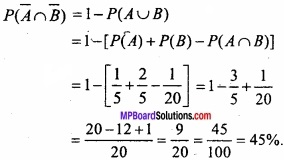Question 2.
A speaks truth in 75% of the cases and B in 80% of the cases. In what percentage of cases are they likely to contradict each other in stating the same fact?
Or
Mohan speaks truth in 75% of cases. Sohan speaks truth in 80% of the cases. In what percentage of cases did the Mohan speaks truth and Sohan speaks lie (or when they contradict each other)?
Solution:
P(A) = Probability that A speaks the truth = $$\frac{75}{100}$$ = $$\frac{3}{4}$$
and P(B) = Probability that B speaks the truth = $$\frac{80}{100}$$ = $$\frac{4}{5}$$
∴ P( $$\bar { A }$$ ) = Probability that A does not speak the truth = 1 – $$\frac{3}{4}$$ = $$\frac{1}{4}$$
and P( $$\bar { A }$$ ) = Probability that B does not speak the truth = 1 – $$\frac{4}{5}$$ = $$\frac{1}{5}$$
It is clear that A and B will contradict each other if one of them speaks the truth and the other does not.
∴ P(A and B contradict) = P(A) P( $$\bar { B }$$ ) + P( $$\bar { A }$$ ) P(B)
= $$\frac{3}{4}$$ × $$\frac{1}{5}$$ + $$\frac{1}{4}$$ × $$\frac{4}{5}$$ = $$\frac{7}{20}$$ = $$\frac{35}{100}$$
Hence, in 35% cases, A and B will contradict each other.Question 3.
Prove that P(A) + P( $$\bar { A }$$ ) = 1?
Solution:
In total a + b trails, if an events can happen in a ways and fails in b ways, when all of these ways being equally likely to occur, then the probability of happening of event A,
P(A) = $$\frac { a }{ a+b }$$
and probability of failing of event A,
P( $$\bar { A }$$ ) = $$\frac { b }{ a+b }$$
Adding eqns. (1) and (2), we get
P(A) + P( $$\bar { A }$$ ) = $$\frac { a }{ a+b }$$ + $$\frac { b }{ a+b }$$
= $$\frac { a+b }{ a+b }$$ = 1.
∴ P(A) + P( $$\bar { A }$$ ) = 1. Proved.

Question 4.
If P(A) = $$\frac{1}{2}$$, P(B) = $$\frac{1}{9}$$ and P(A∩B) = $$\frac{1}{18}$$, then find the value of following:

(i) P( $$\frac{A}{B}$$ ),

(ii) P( $$\frac{B}{A}$$ )

(iii) P(A∪B)

Solution:
Given: P(A) = $$\frac{1}{2}$$, P(B) = $$\frac{1}{9}$$ and P(A∩B) = $$\frac{1}{18}$$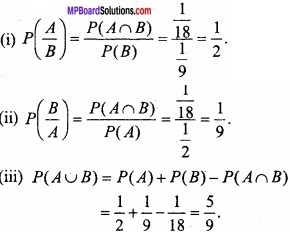Question 5.
A group of 10 children contains 6 boys and 4 girls. Three children are chosen at random from this group. Find the probability that this group chosen:

1. Does not contain any girl.
2. Contains at least one girl.

Solution:
Total number of children = 6B + 4G = 10
3 children out of total 10 may be chosen in 10C3 ways

1. 3 boys out of 6 boys may be chosen in 6C3 ways
∴ Required probability = $$\frac { ^{ 6 }C_{ 3 } }{ ^{ 10 }C_{ 3 } }$$ = $$\frac{1}{6}$$

2. At least 1 girl may be taken as follows
2 boys + 1 girl, number of ways = 6C2 × 4C1
or 3 girls, number of ways = 4C3
Hence, the required probability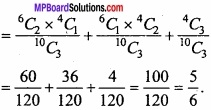Question 6.
4 cards are fallen down by one during the shuffling of the cards. Find the probability that one card is heart, the other is diamond, the third is spade and the fourth is a club?
Solution:
Number of cards = 52
The first can fall in 52 ways.
The favourable ways for the first card to be heart = 13
Probability = $$\frac{13}{52}$$ = $$\frac{1}{4}$$
The number of remaining cards = 52 – 1 = 51
Another card can be fall in 51 ways.
The favourable ways for this card to be a spade = 13
Probability = $$\frac{13}{50}$$
Number of remaining cards = 50 – 1 = 49
∴ Probability = $$\frac{13}{49}$$
Therefore, by compound probability principle, required probability
= $$\frac{1}{4}$$ × $$\frac{13}{51}$$ × $$\frac{13}{50}$$ × $$\frac{13}{49}$$Question 7.
A problem of Maths is given to three students whose chances of solving it are $$\frac{1}{2}$$, $$\frac{1}{3}$$ and $$\frac{1}{4}$$. What is the probability that the problem is solved by all?
Solution:
Let the probabilities of solving the question by the three students be P1, P2, P3 respectively.
Then given that P1 = $$\frac{1}{2}$$, P2 = $$\frac{1}{3}$$, P3 = $$\frac{1}{4}$$
∴ The probabilities of not solving the problem by them are
q1 = 1 – p1 = 1 – $$\frac{1}{2}$$ = $$\frac{1}{2}$$
q2 = 1 – p2 = 1 – $$\frac{1}{3}$$ = $$\frac{2}{3}$$
and q3 = 1 – p3 = 1 – $$\frac{1}{4}$$ = $$\frac{3}{4}$$
∴ Probability that all of the three students do not solve the problem
= q1q2q3
= 1 – $$\frac{1}{4}$$ = $$\frac{3}{4}$$.

Question 8.
A bag contains 6 red, 4 white and 5 blue balls. If balls are drawn one by one from bag and they are not replaced, then what is the probability of 1st red, 2nd white and 3rd blue?
Solution:
A bag contains 6 red (R), 4 white (W), 5 blue (B) balls.
Total No. of balls = 6 + 4 + 5 = 15
∴ Probability of 1st ball is red,
P(R) = $$\frac{6}{15}$$
Now total balls in the bag 15 – 1 = 14
∴ Probability of 2nd ball is white,
P(W) = $$\frac{4}{14}$$
Now the total No. of balls in bag 14 – 1 = 13
∴ Probability of 3rd ball is blue,
P(B) = $$\frac{5}{13}$$
Hence, required probability
= $$\frac{6}{15}$$ × $$\frac{4}{14}$$ × $$\frac{5}{13}$$
= $$\frac{2}{5}$$ × $$\frac{2}{7}$$ × $$\frac{5}{13}$$ = $$\frac{4×1}{91}$$ = $$\frac{4}{91}$$

Question 9.
A bag contains 6 red, 4 white and 6 blue balls. If balls are drawn one by one from bag without replacement, then what is the probability of drawing 1st ball red, 2nd ball white and 3rd ball blue?
Solution:
Solve as Q. No. 8.Question 10.
Two cubical dice are thrown simultaneously. Find the probability that the first dice shows an even number or both the dice show the sum 9?
Solution:
Here (S) = 6 × 6 = 36
The events of getting an even number on the first dice is
E1 = {(2, 1), (2,-2), (2, 3), (2,4), (2, 5), (2, 6), (4, 1), (4, 2), (4, 3), (4,4), (4, 5), (4, 6), (6, 1), (6, 2), (6, 3), (6,4), (6, 5), (6, 6)}
∴ n(E1) = 18
The event of getting a total of 9 on the two dice is
E2 = {(3,6), (4, 5), (5,4), (6, 3)}
∴ n(E2) = 4 .
Also, E1∩E2
⇒ n(E1∩E2) = 2
∴ P(E1) = $$\frac{18}{36}$$, P(E2) = $$\frac{4}{36}$$ and P(E1∩E2) = $$\frac{2}{36}$$
∴ The required probability = P(E1∪E2)
= P(E1) + P(E2) – P(E1∩E2)
= $$\frac{18}{36}$$ + $$\frac{4}{36}$$ – $$\frac{2}{36}$$ = $$\frac{20}{36}$$ = $$\frac{5}{9}$$Question 11.
Out of two bags one contains 3 black and 4 red balls and the second bag contains 8 black and 10 red balls. If one bag is chosen and a ball is drawn from it, then find the probability that is a red ball?
Solution:
I bag
3B + 4R = 7 balls total

II bag
8B + 10R = 18 balls total
(i) Selecting the I bag:
Probability that one bag is chosen (out of two bags) = $$\frac{1}{2}$$
1 red ball is drawn from I bag probability = $$\frac{4}{7}$$
Hence, the probability that the I bag is chosen as well as 1 red ball is drawn from it
(Compound event) P1 = $$\frac{1}{2}$$ × $$\frac{4}{7}$$ = $$\frac{2}{7}$$ ………………… (1)

(ii) If selecting II bag:
Probability that Il bag is chosen = $$\frac{1}{2}$$
I red ball is drawn from II bag probability = $$\frac{10}{18}$$ = $$\frac{5}{9}$$
Hence, the probability that the II bag is chosen as well as 1 red ball is drawn from it
(Compound event) P2 = $$\frac{1}{2}$$ × $$\frac{5}{9}$$ = $$\frac{5}{18}$$ ……………………… (2)
The above two event are mutually exclusive.
Hence, only one event out of these two will happen.
Hence, the required probability P = P1 + P2
= $$\frac{2}{7}$$ + $$\frac{5}{18}$$
= $$\frac{36+35}{126}$$ = $$\frac{71}{126}$$.Question 12.
There are two bags, one contains 5 red and 7 white balls and second bag contains 3 red and 12 white balls. One ball is drawn from any of these bags at random? Find the probability that the ball is red?
Solution:
Solve same as Q. No. 11.

Question 13.
A bag contains 50 bolts and 150 nuts. Half of the bolts and nuts are rusted. If one item is taken out at random find out the probability that ¡ts rusted o is a bolt?
Solution: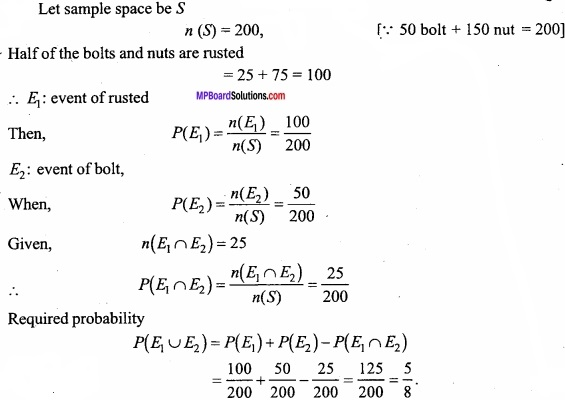Question 14.
A can hit a target 4 times in 5 shots; B, 3 times in 4 shots and C, 2 times 3 shoots at one time. Find the probability of at least two shots hit?
Solution:
Probability of A hitting the target = $$\frac{4}{5}$$
Probability of B hitting the target = $$\frac{3}{4}$$
Probability of C hitting the target = $$\frac{2}{3}$$
At least 2 can hit the target in the following ways:
(i) Probability that A, B,C all hit the target = $$\frac{4}{5}$$ × $$\frac{3}{4}$$ × $$\frac{2}{3}$$ = $$\frac{2}{5}$$

(ii) Probability that B and C hit the target and not A
= ( 1 – $$\frac{4}{5}$$ ) × $$\frac{3}{4}$$ × $$\frac{2}{3}$$ = $$\frac{1}{5}$$ × $$\frac{3}{4}$$ × $$\frac{2}{3}$$ = $$\frac{1}{10}$$

(iii) Probability that C and A hit the target and not B
= $$\frac{4}{5}$$ × (1 – $$\frac{3}{4}$$ × $$\frac{2}{3}$$ = $$\frac{4}{5}$$ × $$\frac{1}{4}$$ × $$\frac{2}{3}$$
= $$\frac{2}{15}$$

(iv) Probability that A and B hit the target but not C
= $$\frac{4}{5}$$ × $$\frac{3}{4}$$ × (1 – $$\frac{2}{3}$$) = $$\frac{4}{5}$$ × $$\frac{3}{4}$$ × $$\frac{1}{3}$$ = $$\frac{1}{5}$$
Since, all these events are mutually exclusive,
∴ Required probability = $$\frac{2}{5}$$ + $$\frac{1}{10}$$ + $$\frac{2}{15}$$ + $$\frac{1}{5}$$ = $$\frac{5}{6}$$Question 15.
In a class 30% students fail in physics, 25% fails in maths and 10% fails in both. If one student selects at random, then And the probability of:

(i) Fail in maths when fail in physics also,
(ii) Fail in physics when fail in maths also,
(iii) Fail in maths or physics.

Solution:
Probability of fail in physics is
P(A) = $$\frac{30}{100}$$
Probability of fail in maths is
P(B) = $$\frac{25}{100}$$
Probability of fail in physics and maths both is
P(A∩B) = $$\frac{10}{100}$$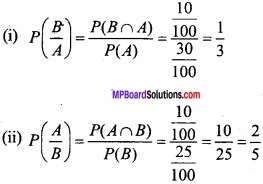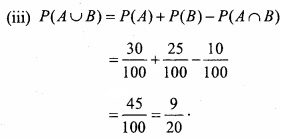Question 16.
Two bags A and B contain 8 green and 9 white balls and 5 green and 4 white balls respectively. One ball is drawn at random from one of the bags and it is found to be green. Find the probability that it is drawn from bag B? (NCERT)
Solution:
Let E1 : Event of choosing bag A
∴ P(E1) = $$\frac{1}{2}$$ ………………………. (1)
E2 = $$\frac{1}{2}$$ ………………………… (2)
Again, let C: Event of drawing a green ball
∴ By defnition of conditional probability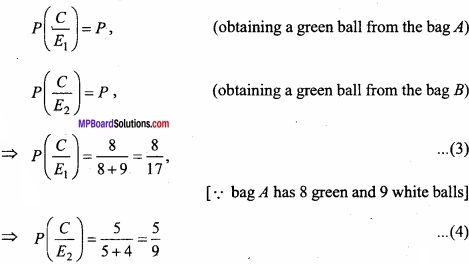The required probability (i.e; the probability of obtaining a ball from bag B when it is green.)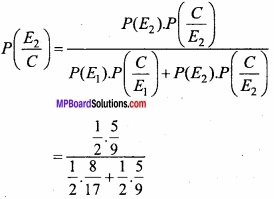Question 17.
A company has two plants to manufacture bicycles. The first plant manufacture 60% of the bicycles and second plant 40%. Also 80% of the bicycles are rated standard quality at the first plant and 90% of standard quality at the second plant. A bicycle is picked up at random and found to be standard quality? Find the probability that it comes from the second plant? (CBSE 2003)
Solution:
Let E1: The event of choosing a bicycle from first plant.
∴ P(E1) = $$\frac{60}{100}$$ ………………….. (1)
Let E2: The event of choosing a bicycle from first plant.
P(E2) = $$\frac{40}{100}$$ ……………………….. (2)
Let E be event of choosing a bicycycle of standard quality, then
P ( $$\frac { E }{ E_{ 1 } }$$ ) = Probability of choosing a bicycle of standard quality, given that it is produced by the first plant.
⇒ P ( $$\frac { E }{ E_{ 1 } }$$ ) = $$\frac{80}{100}$$, (given 80%)
P( $$\frac { E }{ E_{ 2 } }$$ ) = Probability of choosing a bicycle of standard quality, given that it is produced by the second plant.
⇒ P( $$\frac { E }{ E_{ 2 } }$$ ) = $$\frac{90}{100}$$ (given 90%)
∴ The required probability (i.e., probability of choosing a bicycle from the second plant, given that it is of standard quality,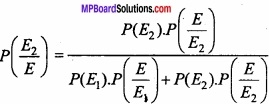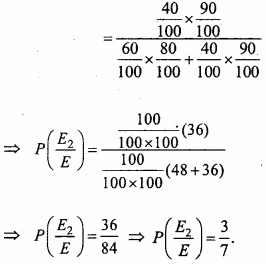Question 18.
A company manufactures T.V., machine A, B and C manufatures 30%, 20% and 50% T.V. of the total production of their outputs 7%, 5% and 2% are defec¬tive T.V. respectively. A T.V. is selected at random from the production and is found to be defective?
Find the probability that defective T.V. which is manufactured by machine A? (CBSE 2015)
Solution:
Let the events T1, T2 and T3 are the following:
Event T1 : T.V. manufactured by machined A.
Event T2 : T.V. manufactured by mechine B.
Event T3: T.V. manufactured by machine C.
It is noted that these events are mutually exclusive.
Again, let E : The event of defective T.V.
Given, P(T1) = 30% = $$\frac{30}{100}$$ ………………….. (1)
P(T2) = 20% = $$\frac{20}{100}$$ ………………….. (2)
P(T3) = 50% = $$\frac{50}{100}$$ ………………….. (3)
Again P( $$\frac { E }{ T_{ 1 } }$$ ) = The probability that T.V. is defective when it is manufactured by machine A.
⇒ P($$\frac { E }{ T_{ 1 } }$$ ) = 7% = $$\frac{7}{100}$$
P( $$\frac { E }{ T_{ 2 } }$$ ) = The probability that T.V. is defective when it is manufactured by machine B.
⇒ P( $$\frac { E }{ T_{ 3 } }$$ ) = 5% = $$\frac{5}{100}$$
Similarly,
P( $$\frac { E }{ T_{ 3 } }$$ ) = The probability that T.V. is defective when it is manufactured by machine C.
⇒ P( $$\frac { E }{ T_{ 3 } }$$ ) = 2% = $$\frac{2}{100}$$
Now, from Bayes’s theorem
P( $$\frac { T_{ 1 } }{ E }$$ ) = The probability that T.V. is defective when it is manufactured by machine A.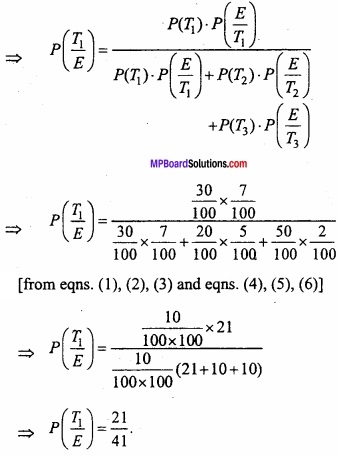Question 19.
The probability that Mohan does not speak the truth is $$\frac{1}{5}$$ Mohan speaks the head when a coin is thrown, find the probability that really gettting head by the throw of a coin? (NCERT)
Solution:
Let A: The event of getting head.
P(A) = $$\frac{1}{2}$$
B: The event of not getting head.
∴ P(B) = 1 – P(B) = 1 – $$\frac{1}{2}$$ = $$\frac{1}{2}$$
Again, Let E: The event that really getting head by the throw of a coin and Mohan speaks.
P( $$\frac{E}{B}$$ ) = $$\frac{1}{5}$$
and P( $$\frac{E}{A}$$ ) = 1 – $$\frac{1}{5}$$ = $$\frac{4}{5}$$
Now, from Bayes’s thoerem
P( $$\frac{A}{E}$$ ) = Probability that the report of the Mohan that head has occured is actually head.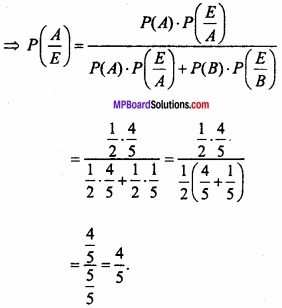Question 20.
Bag A contains 3 white and 4 red balls and bag B contains 5 white and 6 red balls. One ball is drawn at random from one of the bags and it is found to be red. Find the probability that it is drawn from bag B?
Solution:
Let E1 = Event of choosing bag A.
E2 = Event of choosing bag B.
P(E1) = $$\frac{1}{2}$$; P(E2) = $$\frac{1}{2}$$ ………………….. (1)
⇒ P(E1) = P(E2) = $$\frac{1}{2}$$
Again, let R = Event of drawing a red ball.
According to the question.
P( $$\frac { R }{ E_{ 1 } }$$ ) = The probability that a red ball is drawn when it is selected from bag A.
⇒ P( $$\frac { R }{ E_{ 1 } }$$ ) = $$\frac{4}{3+4}$$ = $$\frac{4}{7}$$ …………………. (2) [since bag A contains 3 white and 4 red balls]
P( $$\frac { R }{ E_{ 2 } }$$ ) = $$\frac{6}{5+6}$$ = $$\frac{6}{11}$$ ……………….. (3) [since bag B contains 5 white and 6 red balls]
Now, from Bayes’s theorem,
P( $$\frac { E_{ 2 } }{ R }$$ ) = The probability that a ball is drawn from bag B when it is red.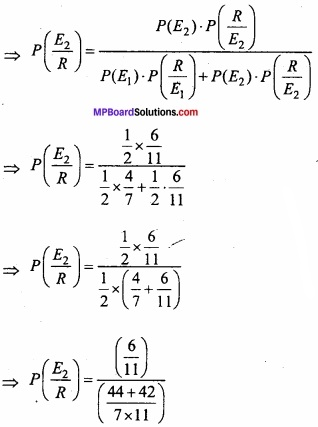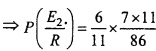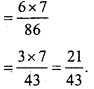Question 21.
Three different bags A, B and C are given, each bag contains 2-2 books. Bag A contains both 2 mathematics books, bag B contains 2 chemistry books and bag C contains, one mathematics and one chemistry book. The student select one bag ran¬domly and from that bag he randomly take out one book. The book he has taken out is that of mathematics. Find the probability that the second book he draws out from that bag is also of mathematics or that he is selected bag A given? (NCERT)
Solution:
Let events E1, E2 and E3 represents the selection of bag A, B and C respctively.
P(E1) = P(E2) = P(E3) = $$\frac{1}{3}$$ …………………….. (1)
D: The event of mathematics book happen
P( $$\frac { D }{ E_{ 1 } }$$ ) = P(A mathematics book chosen from bag A)
= $$\frac{2}{2}$$ = 1 ……………….. (2)
P( $$\frac { D }{ E_{ 2 } }$$ ) = P(A mathematics book chosen from bag B)
= $$\frac{0}{2}$$ = 0 ………………… (3)
[∵according to the questions, both chemistry books are in bag B]
P( $$\frac { D }{ E_{ 3 } }$$ ) = P(A mathematics book chosen from bag C)
= $$\frac{1}{2}$$
Now, the required probability
P( $$\frac { E_{ 1 } }{ D }$$ )
Now, by Bayes’ thoerem,
P( $$\frac { E_{ 1 } }{ D }$$ )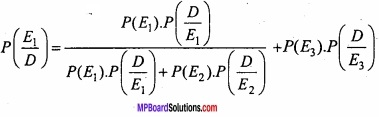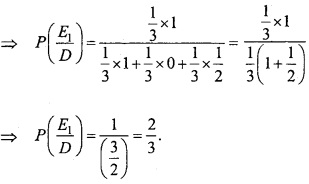Question 22.
By examining about a student that he is known to speak the truth 2 out of 5 times. He throws a die and reports that it is a six. Find the probability that it is actually a six?
Solution:
Let E: Event that the student reports that it is a six.
A : Event of getting a six on the upper face of die.
B : Event of not getting a six on the upper face of die.
∴ P(A) = $$\frac{1}{6}$$ ………………….. (1)
P(B) = P(Not A) = P( $$\bar { A }$$ )
⇒ P(B) = 1 – P(A) = 1 – $$\frac{1}{6}$$
⇒ P(B) = $$\frac{5}{6}$$ ………………… (2)
P( $$\frac{E}{A}$$ ) = Probability that the student reports that six occurs, when six has actually occured.
P ( $$\frac{E}{A}$$ ) = Probability that the student speaks the truth
= $$\frac{2}{5}$$ …………………… (3)
Now, P ( $$\frac{E}{B}$$ ) = Probability that the student reports that six occurs, when six that has not actually occured.
P ( $$\frac{E}{B}$$ ) = Probability that the student does not speak the truth.
P( $$\frac{E}{B}$$ ) = 1 – $$\frac{2}{5}$$ = $$\frac{3}{5}$$
Now, by Bayes’s thoerem
P( $$\frac{A}{E}$$ ) = Probability of getting six, given that the student reports it to be six.